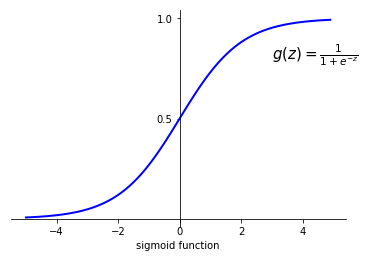# LeetCode Contest 53

class Solution(object):
def hasAlternatingBits(self, n):
"""
:type n: int
:rtype: bool
"""
b = bin(n)[2:]
return all(b[i]!=b[i+1] for i in range(len(b)-1))


# Support Vector Machine

## Intuition

In a binary classification problem, we can use logistic regression

$$h_\theta(x) = \frac{1}{1+e^{-\theta^T x}} = g(\theta^T x),$$

where $g$ is the sigmoid function with a figure of it below.Then given input $x$, the model predicts $1$ if and only if $\theta^x \ge 0$, in which case $h_\theta(x) = g(\theta^T x) \ge 0.5$; and it predicts $0$ if and only if $\theta^T x < 0$. Moreover, based on the shape of sigmoid function, if $\theta^T x >> 0$, we are very confident that $y=1$. Likewise, if $\theta^T x << 0$, we are very confident that $y=0$. Therefore, we hope that for the training set $\{(x^{(i)}, y^{(i)})\}_{i=1}^m$, we can find such a $\theta$ that $\theta^T x^{(i)} >> 0$ if $y^{(i)}=1$ and $\theta^T x^{(i)} << 0$ if $y^{(i)}=0$.

# Generative Model

This article is my notes on generative model for Lecture 5 and 6 of Machine Learning by Andrew Ng. What we do in logistic regression using generalized linear model is that, we approximate $P(y|x)$ using given data. This kind of learning algorithms is discriminative, in which we predict $y$ based on the input features $x$. On the contrary, generative model is to model $P(x|y)$, the probability of the features $x$ given class $y$. In other words, we want to study how the features structure looks like given a class $y$. If we also learn what $P(y)$ is, we can easily recover $P(y|x)$, for example, in the binary classification problem,

$$\begin{equation} P(y=1|x) = \frac{P(x|y=1)P(y=1)}{P(x)}, \label{eqn:bayes} \end{equation}$$

where $P(x) = P(x|y=0)P(y=0) + P(x|y=1)P(y=1)$.

In this article, we are going to see a simple example of generative model on Gaussian discriminant analysis and Naive Bayes.

# LeetCode Contest 52

i = (len(A) + len(B) - 1) / len(A)

class Solution(object):
def repeatedStringMatch(self, A, B):
"""
:type A: str
:type B: str
:rtype: int
"""
i = (len(A) + len(B) - 1) / len(A)
j = 2 * i
ans = -1
while i <= j:
k = (i+j) / 2
tmp = A * k
if tmp.find(B) != -1:
ans = k
j = k - 1
else:
i = k + 1
return ans


# Generalized Linear Model (Examples)

This article is a companion article to my another post Generalized Linear Model. In this article, I will implement some of the learning algorithms in Generalized Linear Model. To be more specific, I will do some examples on linear regression and logistic regression. With some effort, google search gives me some very good example data sets to work with. The datasets collected by Larry Winner is one of the excellent sets, which will be used in the article.

The implementations here use Python. Required 3rd party libraries are:

# LeetCode Contest 51

1. 整数：代表本轮得分
2. "+"：本轮得分为上两轮得分之和
3. "D"：本轮得分为上轮得分的两倍
4. "C"：上轮得分无效。

class Solution(object):
def calPoints(self, ops):
"""
:type ops: List[str]
:rtype: int
"""
points = []
for op in ops:
if op == 'C':
points.pop()
elif op == 'D':
points.append(points[-1]*2)
elif op == '+':
points.append(points[-1]+points[-2])
else:
points.append(int(op))
return sum(points)


# Generalized Linear Model

This article on Generalized Linear Model (GLM) is based on the first four lectures of Machine Learning by Andrew Ng. But the structure of the article is quite different from the lecture. I will talk about exponential family of distributions first. Then I will discuss the general idea of GLM. Finally, I will try to derive some well known learning algorithms from GLM.

## Exponential Family

A family of distributions is an exponential family if it can be parametrized by vector $\eta$ in the form $$P(y; \eta) = b(y)\exp(\eta^{T} T(y)-a(\eta)),$$ where $T(y)$ and $b(y)$ are (vector-valued) functions in terms of $y$, and $a(\eta)$ is a function in terms of $\eta$.

$\eta$ is called the natural parameter and $T(y)$ is called the sufficient statistic.

# LeetCode Contest 49

class Solution(object):
def findLengthOfLCIS(self, nums):
"""
:type nums: List[int]
:rtype: int
"""
ans = i = 0
while i < len(nums):
j = i + 1
while j < len(nums) and nums[j] > nums[j-1]:
j += 1
ans = max(ans, j-i)
i = j
return ans


# LeetCode Contest 48

# Definition for a binary tree node.
# class TreeNode(object):
#     def __init__(self, x):
#         self.val = x
#         self.left = None
#         self.right = None

class Solution(object):
def findSecondMinimumValue(self, root):
"""
:type root: TreeNode
:rtype: int
"""
nums = set()
def dfs(root):
if not root:
return